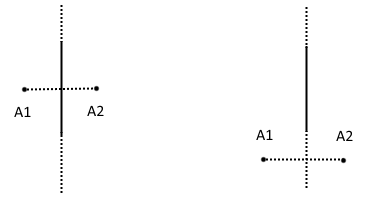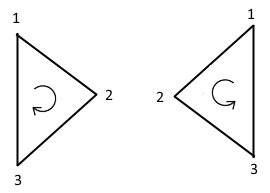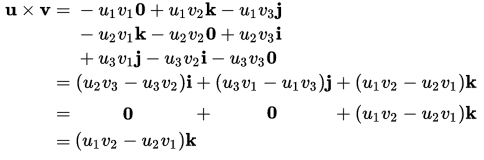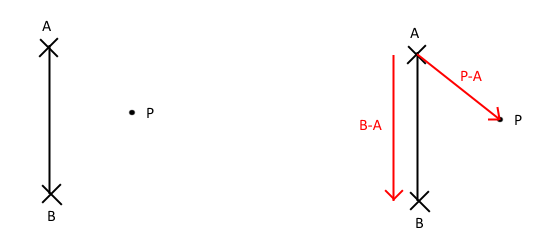# Detecting Line Crossings

I’ve been working on a small game recently, the goal of which is to change your player’s color to match the colors of incoming lines so that you can pass through them. Detecting when the player crosses a line is at the core of the game.

Detecting which side of a 2D line a point is on is a pretty simple task. I’d wager most people have encountered the problem relatively early in their game development efforts (not allowing the player to move outside of the screen, for example). Nonetheless it’s probably something that people (including me) have solved in an inefficient or needlessly complex way. So I’m going to go over the problem and solution implemented in my game.

#### Definition

This problem assumes that the line that we’re checking is functionally infinitely long. That is, it splits the 2D plane. (A more technical explanation is available on Wolfram Alpha). Now, the visible portion or the portion of interest can be a finite length, but mathematically we want to be able to check if a point is on one side of the line that divides the plane or the other.

What we’re not trying to solve is checking if a point crosses “through” an arbitrary length-line.

To illustrate the point:A1 and A2 represent the position of the point of interest at different times (for example, the player’s position). The solid part of the line that’s being crossed represents the part of interest (the visual component being drawn, screen edge, etc). The dashed part of the lines represent the split that is made on the 2D plane that goes on infinitely. In both cases we would define the point’s transition from A1 to A2 as “crossing the line”.

If every line was either vertical or horizontal it would be simple to check if the line was crossed, you would just check the x position for vertical lines or the y position for horizontal lines.

But what about diagonal lines? What if the line is moving? What if the line is moving and rotating? That could really complicate some solutions.

#### Solution

The solution to this problem has its roots in the 3D problem of back-face culling. The TL;DR version of the problem is as follows:

In 3D graphics everything is made of triangles, each of which has three vertices, which we’ll label 1, 2, and 3. We can define “looking at the front” of the triangle to mean that when the triangle is projected onto screen, the vertices are wound either clockwise or counter-clockwise. As a result, “looking at the back” of the triangle would mean the vertices are wound in the opposite direction. If we’re “looking at the back” of the triangle, skip drawing it.

Notice though that if one of the vertices crosses the line that goes between the other two vertices that the winding changes. For example, if vertex 2 is moved to the other side of the line that goes between 1 and 3:That’s the basis of the solution to the line crossing problem, we need to find out when the “triangle” winding changes. In our case the vertices of our triangle are defined as: 1 and 2) Two arbitrary, non-equal, points on the line in question 3) The position of the player.

The problem still exists though that we need a mathematical way to know when the winding changes. Luckily we can adapt an existing mathematical principle for that use, the vector cross product.

Take a look at a visual guide to the cross product:Image taken from WikiMedia Commons

Notice that when the vector b swaps which “side” of a it’s on, the direction of the resultant cross product vector changes. We really aren’t interested in the magnitude of the vector, just its direction, up or down. “But we’re working in 2D, not 3D!” you say. That’s okay, if we examine the equation for the cross product we find that we’ll still get what we want. Here’s the formula for a 3D cross product:If we project our 2D vectors into 3D we can consider the Z values (u3 and v3 in the equation) to be zero. That makes the i and j components of the cross product 0, which just leaves the k component.The k component is what we were looking for. Or more specifically, its sign. Since the k component is the only component that ever has a value we can disregard the fact that the result is a vector and just consider the scalar value of the k component.

To get our u and v vectors we’ll take the offsets of points on the “triangle” we defined earlier. The first vector, u, will be the displacement vector from point 1 on our line (A in the picture) to point 2 on our line (B in the picture). The second vector, v, will be the displacement vector from point 1 on our line to the player’s position (P in the picture).Once we have those vectors we just need to do some simple arithmetic to get the cross product. The sign of which will tell us what side of the line the player is on. This is how the lines in my game keep track of which side the player is on:

```private void FindPlayerSide(Vector2f playerPos)
{
Vector2f endDis = this.Point2 - this.Point1;
Vector2f playerDis = playerPos - this.Point1;
Vector2f cross = endDis.Cross(playerDis);
this._playerSide = cross > 0 ? 1 : -1;
}
```

`Vector2f` is a type provided by the SFML library. The `Cross()` method is an extension method I made for Vector2f objects, which just provides a shorthand way to do the arithmetic previously derived. Here’s the code for that method:

```public static class Vector2FExtensions
{
public static float Cross(this Vector2f vec, Vector2f other)
{
return vec.X*other.Y - vec.Y*other.X;
}
}
```

The end result is that all the line needs to do to know whether the player crossed it or not is to check if the value of `_playerside` has changed. It doesn’t matter how the line is rotated, how long or short the visible component is, or whether the line, player, or both are moving. Which is great because it allows me to use the same code for all cases. Also, all of the math that’s used to accomplish this just makes use of simple addition, subtraction, and multiplication. There’s no square root, trigonometry, or other expensive math operations in play.